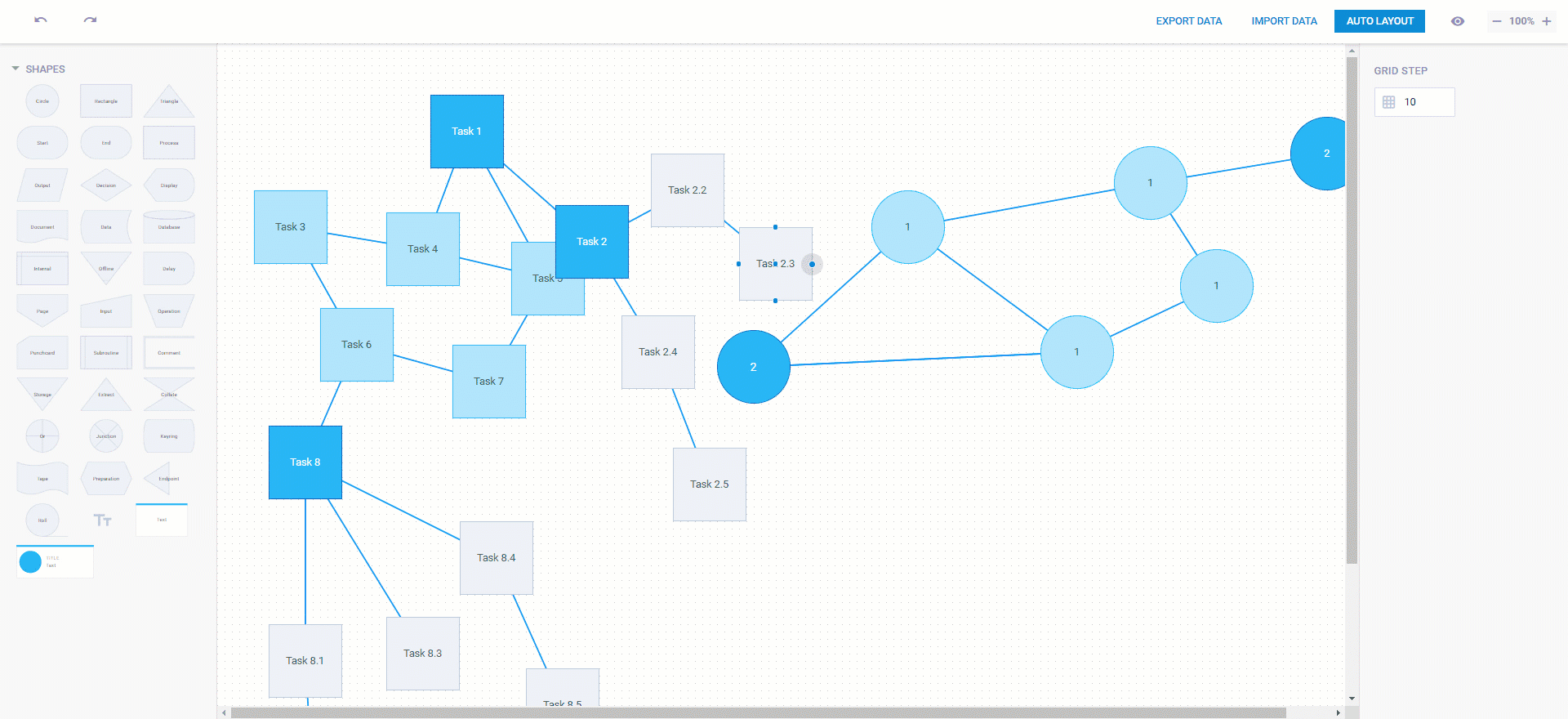# Using Layout Algorithms to Arrange Diagram Shapes Automatically

|

Modern diagramming libraries frequently come with a special tool that helps to speed up the creation of different diagram types – a layout algorithm. This feature is intended for automatic arrangement of diagram elements. Based on specific rules, the algorithm calculates the positioning of diagram shapes and connectors and places them in such a way that even the most complex diagrams become clear and informative.

In this post, we will take a closer look at the most popular auto layout algorithm types, see how they are implemented in DHTMLX Diagram, and consider from a more technical standpoint how web developers can apply the auto layout function using our diagramming component.

##### Types of Automatic Layout Algorithms

Layout algorithms may vary in numerous aspects, but most commonly they differ in approaches to how shapes are interconnected i.e. what kind of connectors are used in the diagram.

Based on this attribute, layout algorithms are divided into many types, but the most popular of them are hierarchical layout and orthogonal layout.

###### Hierarchical Layout Algorithm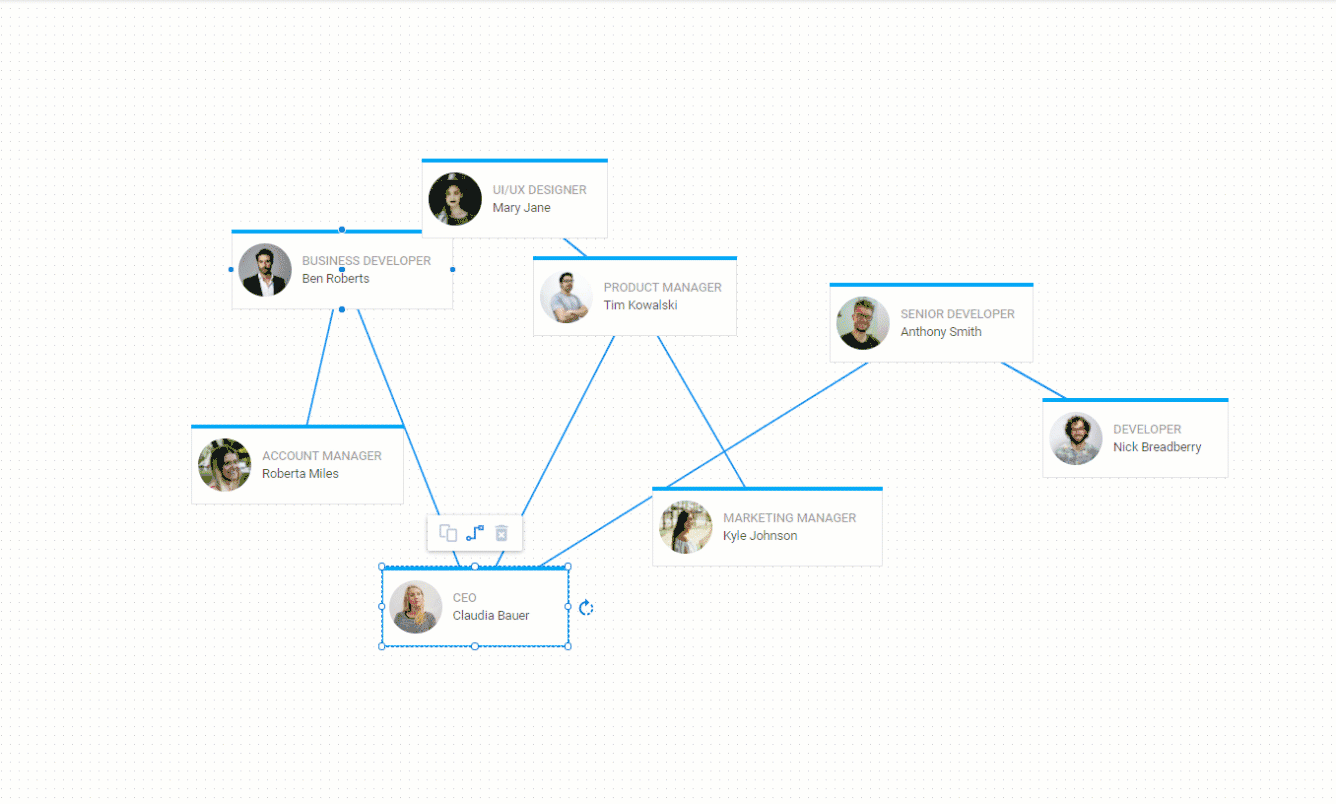Hierarchical layout in the org chart by DHTMLX

A hierarchical layout algorithm uses straight and diagonal connectors for arranging loaded data in a hierarchical order. It helps to clearly show the principal direction (or flow) within a diagram. Here shapes of the diagram are commonly positioned in hierarchically structured layers with most of the connectors oriented in the same direction (left-to-right, top-to-bottom, etc.). Thanks to a small possibility of crossings between connectors, the algorithm brings more clarity to the diagram.

The hierarchical layout algorithm is popular in the application areas where it is necessary to clearly show dependencies between diagram nodes. For instance, it is widely used for visualizing business activities (workflow diagrams, PERT charts, org charts), software engineering (activity diagrams), database modeling, electrical engineering, and other fields with a strong focus on the direction of data flow.

###### Orthogonal Layout Algorithm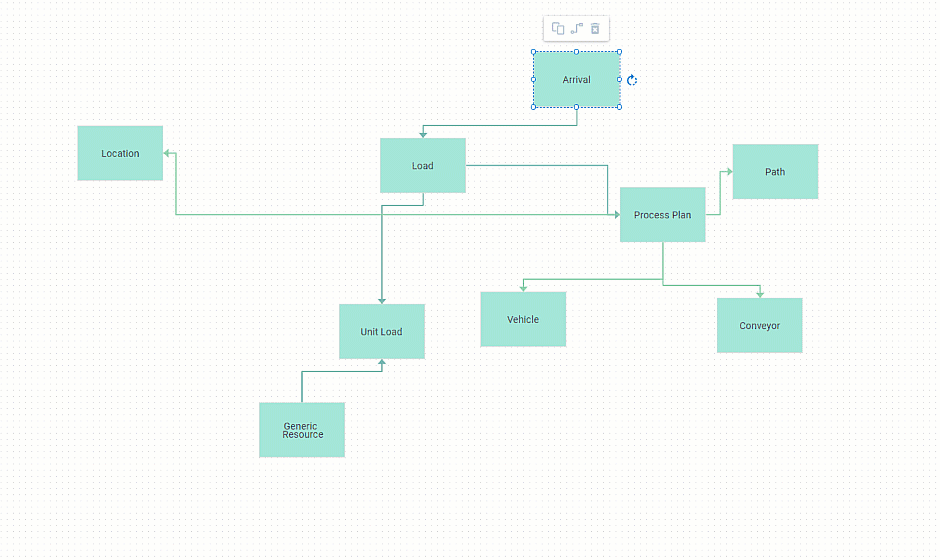Orthogonal layout in the block diagram by DHTMLX

An orthogonal layout utilizes right-angle connectors for presenting directed or undirected graphs allowing you to quickly understand the relationships between nodes. Like the hierarchical layout, the orthogonal layout algorithm helps to minimize the chances of crossings and also ensures a lack of overlaps and as few as possible bends. Thus, diagrams, especially complex ones, become more compact and readable.

An orthogonal layout algorithm is an appropriate option for mid-sized sparse graphs. It can be applied in a database schema, system management, or software engineering. This algorithm can be frequently encountered in ER diagrams or circuit diagrams.

##### How to Use Layout Algorithms in DHTMLX Diagram

When utilizing DHTMLX JavaScript diagram library for data visualization, you can arrange diagram shapes automatically either in the direct mode or in the edges mode. The direct mode allows connecting diagram shapes with diagonal lines in a hierarchical structure. The edges mode engages right-angle connectors and helps you create orthogonal graphs.

In order to configure the automatic layout algorithm, web developers have two possible options:

By default, this method will implement the direct mode of linking shapes.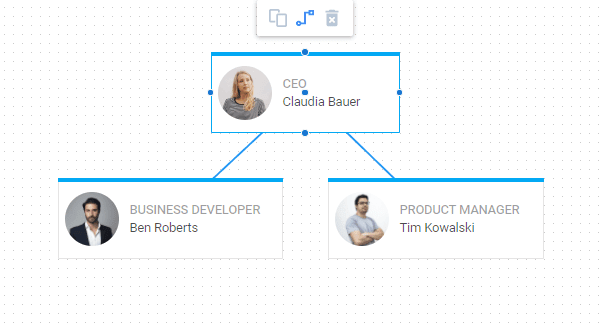Diagonal connectors in the direct mode

Optionally, you can change it to the edges mode via the mode parameter.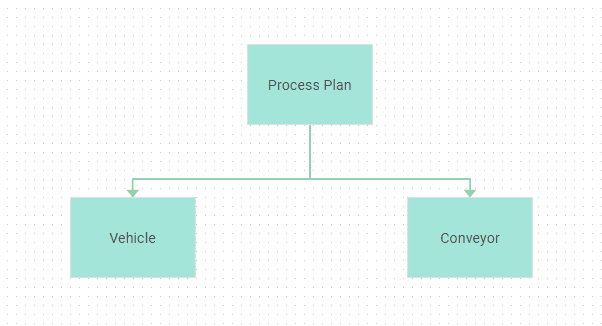Right-angle connectors in the edges mode

If you want to place several diagrams next to each other on the grid, you can apply the graphPadding parameter that determines the interval between diagrams (200px, by default).

var diagram = new dhx.Diagram("diagram");
diagram.data.parse(data);

diagram.autoPlace({
mode: "edges",
});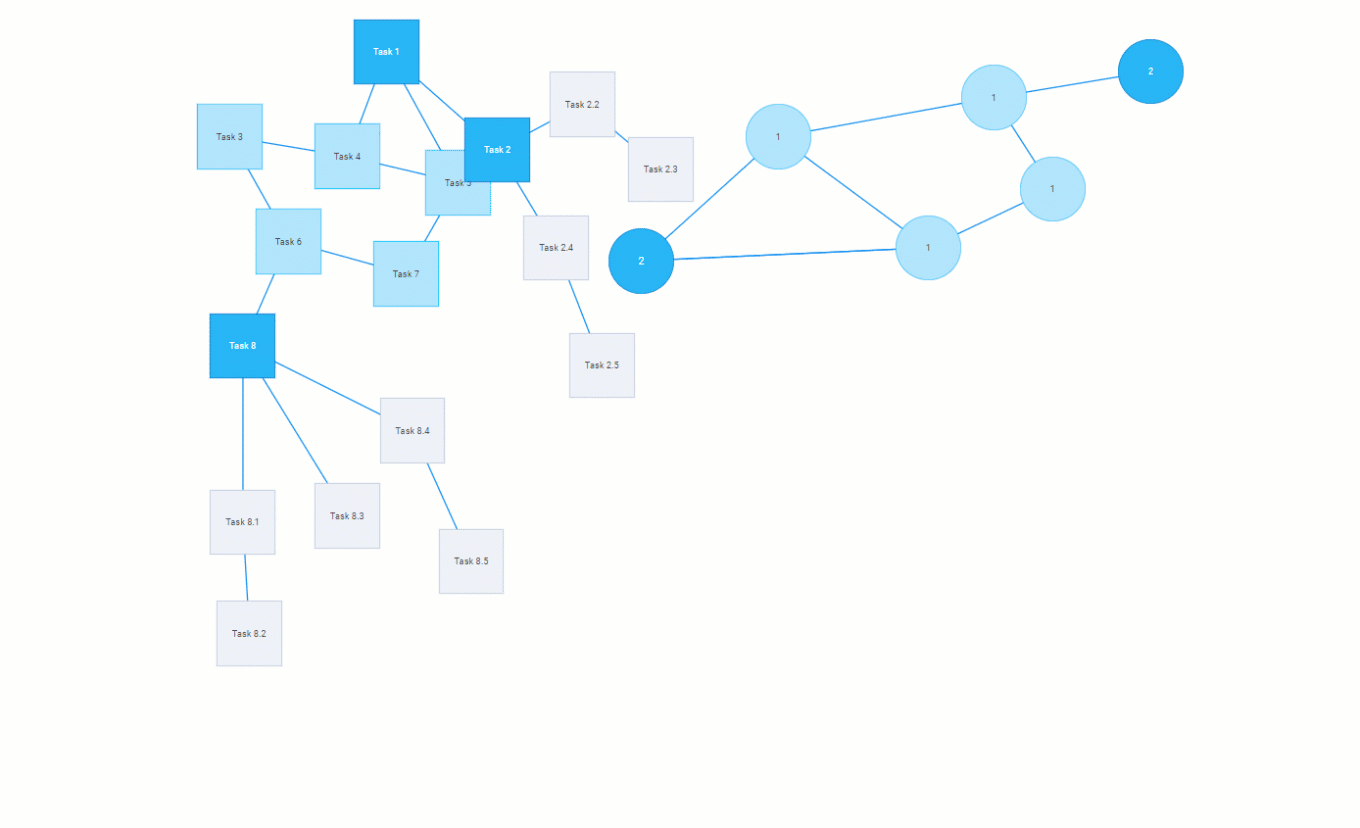Auto layout in the edges mode

• Set the same configuration parameters using the autoplacement property
var diagram = new dhx.Diagram("container", {
autoplacement: {
mode: "edges",